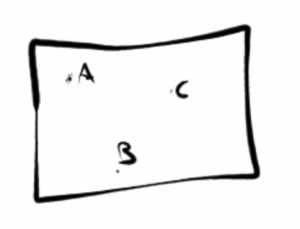# Electric Fields (Part 1 of 2)

Part 2 of Electric Fields is here: Electric Fields (Part 2 of 2)

• Like charges repel
• Opposite charges attract

## Coulomb’s Law (Inverse Square Law)

The magnitude of the force between two charges is proportional to the product of the magnitude of the charges.

Fe = K q1 q2 / r2

q1 × q2 = c2, after multiplication

r2 = m2

| number | – denotes the numbers absolute value – ignoring negative notation, eg. | -3.124 | = 3.124

Ke = approx. 9×109 Nm2/c2

Example:

Q1 = 5×10-3 c

Q2 = -1×10-1 c

∴ Fe = 9×109 Nm2/c2 | (5×10-3 c) (-1×10-1 c) | / (0.5 m)2

Fe = 45×105 Nm2 / 0.25 m2

Fe = 1.8×107 N – Attractive force

Sometimes the r2 is denoted as d2 – for distance

## Conservation of Charge Law

*q = Constant

* = No net change incoming or outgoing

∑ = Sum of all

q = charge (c)

For example, a closed box:• A = +2 c
• B = +5 c
• C = -3 c

The sum of all charges = + 4 c, which remains constant if the box is closed.

These charges may move, collide, exchange charges – the point is: even if the particles change after x amount of time; the charge will still be the same.

To continue with the above box, after 1 hour:

• A = +1 c
• B = -7 c
• C = ?

A + B + C = + 4 c

1 + (-7) + C = + 4 c

∴ C = + 10 c

This law is also true in forms of decaying particles